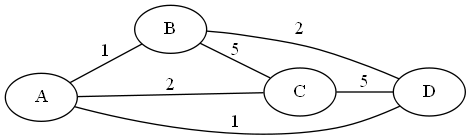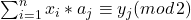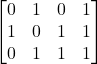# Monthly Archives: September 2023

## Clustering

I feel a little silly I didn’t come up with this one myself.

The problem: Clustering.  This is problem MS9 in the appendix.

The description: Given a complete weighted graph G=(V,E), with a positive weight d(i,j) for each edge eij, and two positive integers K and B.  Can we partition V into K (or less) disjoint sets V1..Vk such that within each Vi, all edges that stay within the partition cost B or less?

Example: Here is a graph:If K=2, and B=2, we could have the sets {A,C} and {B,D} as a legal cluster.  But if  K=2 and B=1, we will not be able to solve the problem.  For example, we might want to have the edges (A,B) and (A,D) in the same cluster, but that would require us also to consider the edge (B,D), which is larger than 1.

Reduction: Brucker’s paper introduces the problem using a graph, like I did above. (G&J talk about points and distances), His reduction is from Graph Coloring.

Suppose we have a coloring graph G.  We build a new graph G’ such that the weight of an edge in G’ is 1 if it did not appear in G, and its weight is 2 if it did appear in G.  Then we set out K = the K of the coloring problem (3 for 3-coloring), and B = 2.  A partition of the vertices into 3 sets where each set has no weight-2 edges is exactly a legal coloring.

Difficulty: 3.  I do think the graph terminology helps to make the problem more understandable.

## Shapley-Shubik Voting Power

Setting up this definition will take a bit, bear with me.

The problem: Shapley-Shubik Voting Power.  This is problem MS8 in the appendix.

The description: Suppose we have a set of n voters, each voter i has a “weight” wi, corresponding to their number of votes.  If we are doing a simple majority vote, a coalition of voters then needs votes of (strictly) more than half the sum of the weights to win.  For a specific voter i, we can look at all permutations of voters, and say that all voters before voter i in the permutation voted “yes”, and all voters after voter i in the permutation voted “no”.  We are interested in the number of these permutations where voter i’s vote “matters”- where adding voter i to the “yes” votes makes “yes” the majority, but adding them to the “no” votes makes “no” the majority.  We call voter i a pivot player if this is the case.

Is voter 1 (in the G&J definition, it’s voter N in the paper) ever a pivot?

Some clarifications:  The number of times a voter is a pivot is the “Shapley-Shubik voting power”, and the proportion of permutations the voter is a pivot (by dividing the count by N!) is the “Shapley-Shubik power index”, but all we care about here is whether the power is non-zero.

Also, the definition of the voting game (in G&J, and also in the paper) allows for a more general definition of winning, besides a simple majority- you can supply a “quota” q, and any amount of votes ≥ q is a winning coalition.  For us, q is set in the reduction to be 1/2 the sum of the weights, +1, rounded up.

Example: Suppose we had 4 voters, with weights: 5.4,3,1.  There is 13 total weight, and 7 weight is enough to win. Voter 4 has 0 coalitions in which they are the pivot (we would need 6 votes without them), so has “zero Shapley-Shubik voting power”.

If, however, we split up the same number of votes among 5 voters: 4,3,3,2,1, then the 1-vote voter can be a pivot with (among others) the 4 and the 2.  The coalition loses without our pivot voter but wins with them.

Reduction: G&J have this as an “unpublished results” problem, but luckily I found a paper by Matsui and Matsui who solved it.  So we start with Partition.  We’re given a set A of n integers.  We will create a set of weights with one weight equal to each element of A, and add one extra voter with weight 1 at the end.  This is the voter that may or may not be a pivot.

If this last voter is a pivot, then there is a coalition that needs an additional weight of 1 to become a majority.  This means the coalition had exactly half of the votes without this voter (and so the sum of the elements is a partition of A)

In the other direction, if the set has a partition, then the partition set’s votes is equal to exactly half of the votes, one short of a majority.  Adding our 1-weight voter will make it a majority, and thus that voter is a pivot.

Difficulty: 4.  This problem looks scary because the definitions of Shapley-Shubik voting power include a lot more than what you actually need to do this reduction.  Once you get past all of that, it’s actually a pretty nice problem that students can handle.

## Decoding of Linear Codes

I think I can get through the rest of the appendix this semester.  Fingers crossed.

The problem: Decoding of Linear Codes.  This is problem MS7 in the appendix.

The description: We are given an NxM boolean matrix A, a vector y with m 0’s and 1’s in it, and an integer K.  Does there exist a boolean vector x, with n elements, and at most K 1’s, where for each column j:?

Example: Here is a matrix A:If our vector y is (1,0,0,1), we are saying we want a vector x that is different from the first and fourth column of A in one (or 3) positions and is different from the second and third column in 0 (or 2) positions.   A vector such as (0,1,1) will do this.

If y was (1,1,1,1), I don’t think it’s possible to come up with any vector x, because since the first two columns of A are opposites, any vector that differs in the first column in 1 (or 3) positions will differ in the second column in 2 (or 0) positions, so we won’t be able to satisfy both elements of y simultaneously.

Reduction:

The paper by Berlekamp, McEliece, and van Tilborg calls this the  “Coset Weights” problem, and uses 3DM, adding the assumption that all 3 dimensions come from the same set of parent elements. So we start with a set U (they call it “W” in the paper) of triples.

We will use these triples to build our matrix A.  Our matrix will have one row for each triple and 3 columns for each element in our parent set (so 3q columns total).  We then build an incidence matrix of each of our triples- each row will have exactly three 1’s in it, corresponding to the element in each of the three dimensions it is.  So, for example, if the sets we are making our triples from were all {1,2,3,4}, and our triple was (1,2,3), the row for that triple would be {1,0,0,0, 0,1,0,0,0,0,1,0}

We’ll choose y to be a vector of all 1’s.  Now we are looking for a vector x of 0’s and 1’s.  This will be like choosing triples from the 3DM instance.  (A 1 in the vector means we choose that row and a 0 means we don’t).  We’ll set K=q to make sure that we choose exactly q triples.

So, a set of q triples that covers all elements will have a 1 in each column in one of these triples and give us a solution to our problem. Similarly, a vector with q 1’s in the correct places that covers all columns will generate our y vector.

Difficulty: 5.  Matrix manipulation is something I personally don’t feel terribly comfortable with, but this is a pretty straightforward implementation of it.